# Introduction to Options and Option pricing using "quantsbin" library¶

## PART 1¶

In this part of te tutorial we will explore below topics:

1. Installing Quantsbin
2. Defining Option objects
3. Calculating and plotting payoff
4. Creating engine object to pass market data to option object
5. Selecting model for valuation
6. Calculating and plotting option premium and greeks



For full set of features, please look at Quantsbin Documentation page

### Installation and Import¶

Let's start with installing Python open source library Quantsbin: Quantsbin library could be installed using either of the below method -

    1. PIP installation: Simply type <pip install quantsbin> in your terminal



Once Quantsbin is installed, import the Derivative pricing module and assign qbdp as alias to it.

In :
import quantsbin.derivativepricing as qbdp


### Defining Option Objects¶

In current release Quantsbin provide options to define under four asset classes. Options on:

1. Equity: qbdp.EqOption
2. Futures: qbdp.FutOption
3. Currencies: qbdp.FXOption
4. Commodities: qbdp.ComOption



In :
print(qbdp.EqOption.__doc__)

    Defines object for vanilla options on equity with both European and American expiry type.

Args required:
option_type: 'Call' or 'Put' (default value is set to 'Call')
expiry_type: 'European' or 'American' (default is set to 'European')
strike: (Float in same unit as underlying price) e.g. 110.0
expiry_date: (Date in string format "YYYYMMDD") e.g. 10 Dec 2018 as "20181210"
derivative_type: Default value as "Vanilla Option".



We can observe above that therevare five argumnents which are required for deifning an option.

Let's deifne our own Equity call option with European expiry.

In :
equity_option1 = qbdp.EqOption(option_type='Call', strike=50, expiry_date='20190621')


Congrates!!! you have defined you first option. Let's explore more into this option.

We will start with calculating payoff given Spot (current underlying price) is 55.

In :
print(equity_option1.payoff(55))

5


### Plotting Payoff¶

Let's plot the payoff profile for this option.

qbdp.Plotting takes first argument as option object, second argument as parameter to be plotted and this argument as range for which plot is required.

Payoff Plotting

In :
eq1_payoff = qbdp.Plotting(equity_option1,'payoff',x_axis_range=[25,75]).line_plot()
eq1_payoff.show()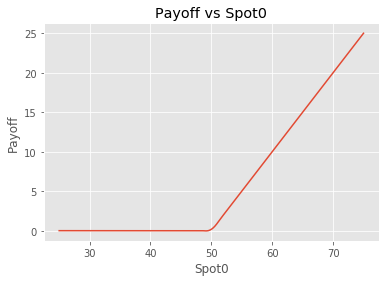### Option Pricing and Greeks Calculation¶

To price option we need two data points: Pricing Model and Market data. We pass these two parameters to our option using engine method which returns engine object.

To start with we are using BlackScholesMerton as our pricing model and updating all other market parameters in engine.

In :
eq1_engine = equity_option1.engine(model='BSM',pricing_date='20180620',spot0=55, rf_rate=0.05, volatility=0.25)


In :
print(eq1_engine.valuation())

9.66280048534


Calculating Option Greeks

In :
print(eq1_engine.risk_parameters())

{'delta': 0.75995706023859544, 'gamma': 0.022580254309896741, 'theta': -0.01025008645649733, 'vega': 17.123101752878206, 'rho': 32.222878479364951, 'phi': -41.912152390692953}


We can also check what all models are available for valuation by using below command

In :
print(equity_option1.list_models())

BSM, MC_GBM, Binomial


Once we have engine object ready, we can start by plotting pnl profile. PnL is simply payoff minus current option premium

Note: We need to pass pricing object for pnl plot, unlike payoff plot where we pass option object

PnL Plotting

In :
eq1_pnl = qbdp.Plotting(eq1_engine, 'pnl', x_axis_range=[25,75]).line_plot()
eq1_pnl.show()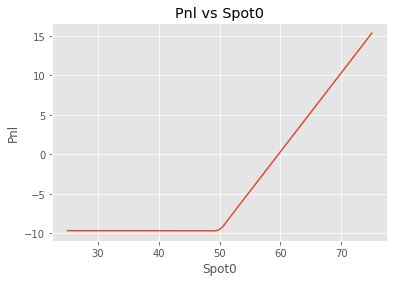In :
eq1_payoff = qbdp.Plotting(equity_option1,'payoff',x_axis_range=[25,75]).line_plot()
eq1_pnl = qbdp.Plotting(eq1_engine, 'valuation', x_axis_range=[25,75]).line_plot()
eq1_pnl.show()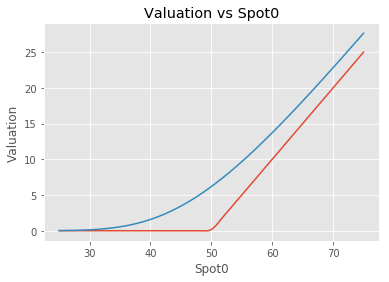Plotting Greeks

Delta

In :
eq1_delta = qbdp.Plotting(eq1_engine, 'delta', x_axis_range=[25,75]).line_plot()
eq1_delta.show()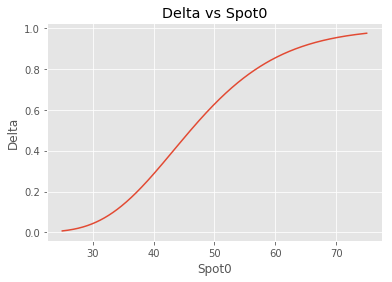Gamma

In :
eq1_delta = qbdp.Plotting(eq1_engine, 'gamma', x_axis_range=[25,75]).line_plot()
eq1_delta.show()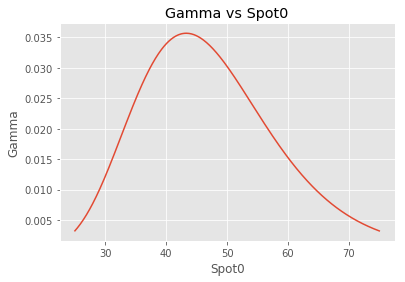Theta

In :
eq1_delta = qbdp.Plotting(eq1_engine, 'theta', x_axis_range=[25,75]).line_plot()
eq1_delta.show()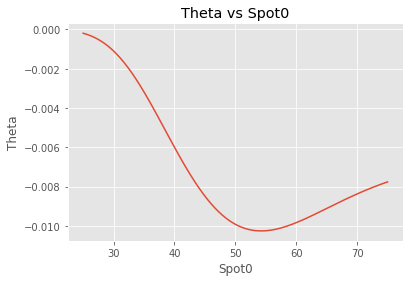Vega

In :
eq1_delta = qbdp.Plotting(eq1_engine, 'vega', x_axis_range=[25,75]).line_plot()
eq1_delta.show()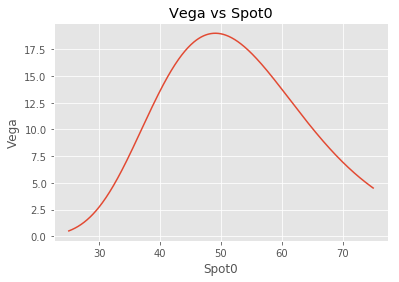Rho

In :
eq1_delta = qbdp.Plotting(eq1_engine, 'rho', x_axis_range=[25,75]).line_plot()
eq1_delta.show()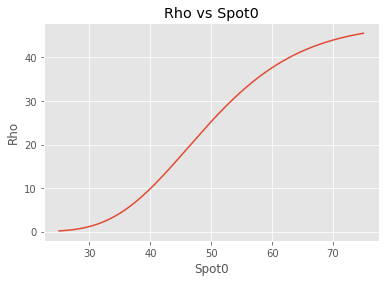In next tutorial we will further deep dive into valuation of options on different asset classes and available market parameters to tweek